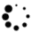# Optimize product blending using Excel spreadsheets and Lingo software—Part 2

Linear programming (LP) for blending. LP is an optimization model that can be used to good advantage despite the highly nonlinear characteristics of the fluid flow-cash flow model.

## Linear programming (LP) for blending

LP is an optimization model that can be used to good advantage despite the highly nonlinear characteristics of the fluid flow-cash flow model. These nonlinearities can be resolved within the framework of a linear program model by adding constraints to make the model piece-wise linear. This requires a powerful computer to solve such linear program models.

The structure of the linear program model closely follows the schematic in FIG. 5. For instance, each refinery is represented by a sublinear program model of 100 or more constraints. Furthermore, sublinear models are used to represent the movement of crude oil from the fields to refineries, and the transportation of finished products from refineries to market.

Areas where this technique is employed include:3

1. Blending gasolines
2. Refinery models
3. Allocation of transportation facilities for shipping products from refineries to terminals
4. Integrated operations, simultaneously considering complete refinery models (2) and transportation models (3)
5. Allocation of transportation facilities for shipping products from the crude oil field to refineries
6. Integrated operations encompassing 1–5.

The general linear programming problem is to find a vector (x1, x2, …xn), where n is the independent variable, which minimizes or maximizes the linear form or the objective function, P as (Eq. 39):

P = c1x1 + c2x2 + c3x3 + ............... cjxj + ............... + cnxn                                      (39)

subject to the linear constraints (Eq. 40):

xj ≥ 0,j = 1,2, ..............., n                                                                                     (40)

and (Eqs. 41–43):

a11x1 + a12x2 + ............... aijxj + ............... + alnxn ≤ bi                                           (41)

ailx1 + ai2x2 + ............... aijxj + ............... + ainxn ≤ bi                                             (42)

amlx1 + am2x2 + ............... amjxj + ............... + amnxn ≤ bm                                      (43)

where aij, bi and ci are given constants and m < n.

One way of looking at the model is that the structure of the fluid-flow portion is represented by the following constraints (Eqs. 44 and 45):

A × X = b                                                                                                                (44)

subject to

X ≥ 0                                                                                                                       (45)

Conversely, the cash flow aspect of the model is represented by minimizing or maximizing C × X, where C = (c1, c2, ... cm) is a row vector, X = (x1, x2, ... xm) is a column vector, A = (aij) is a matrix and B = (b1, b2, ... bm) is a column vector.

The objective function (P) is usually the octane number of the blend that must be maximized. In some instances, the objective function can be the minimized cost or setting the Reid vapor pressure (RVP) to a certain value. The primary constraints variables (x1 to xn) are the volume of each cut of a blend, which must be greater than zero. Other constraints include the capacity of the tank in which the sum of x1,s will not exceed, as well as any blend properties. Therefore, ai1 to amn are the properties or their indices for components 1 to n, and bi to bm are the targeted blending properties of their indices.

In most blending cases, two or more properties are required to be adjusted by the addition of modifiers to the blend. For example, oxygenates are added to enhance gasoline octane number and n-butane is added to adjust the RVP. Determining the quantities of the additives becomes more difficult in non-linear properties cases. Determining the amounts of these blends is based on solving two or more equations with two or more unknowns, depending on the property equations.

A typical refinery optimization problem can be split into sub-problems using a spatial decomposition scheme proposed by Jia and Ierapetritou.4 These sub-problems are solved as independent optimization problems, and the combined results are considered an approximate solution to the overall refinery optimization problem.

Several commercial tools are available for solving stand-alone refinery optimization problems, including software products for online and offline blending optimization problemsa,b; products for online and offline blending optimizationc,d,e; and online optimization solutions for primary and secondary process unitsf,g.

FIG. 6 shows a schematic decomposition of the refinery optimization problem, and FIG. 7 shows an overview of petroleum refining and blending processes.FIG. 6. Schematic division of refinery-wide operation.FIG. 7. Overview of petroleum refining and blending processes.

## Mathematical formulation

The problem formulation includes multiple blenders and storage tanks, as shown in FIG. 6. Properties of the final products are modelled using blend laws that relate the final product property to the quantities and properties of feed components. Blend product properties are either linearly or non-linearly blended based on the volume or mass of the feed components. For example, the RON of the blend is modelled by linear blend laws, while the RVP of the blend is modelled by non-linear blend laws. The blend laws used to estimate product property based on volume/mass fraction or volume/mass flow and property of feed components are:

1. Linearly blended by component fractions in tanks: the property of product blend is a linear function of component volume/mass fractions and properties (Eq. 46):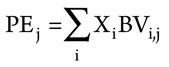(46)
where Xi is the volume/mass fraction of component i in the product tank.
1. Linearly blended by component flows in blend headers: the instantaneous property of the product blend is a linear function of component volume/mass flowrates and properties (Eq. 47):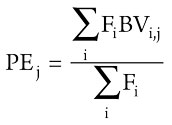(47)
where Fi is the volume/mass flowrate of component i to the blend header.

Blend optimization is subjected to different constraints types, including operational (equipment limits on component flow), inventory (volume limits on feed components) and quality (analyzer limits and tank property specification).

## Solving the problem

Solving the proposed problem in real time is one of the main requirements; however, if this is impossible, then the intermediate iteration result after a fixed time interval should be feasible and better than the previous iteration results. This requirement ensures that a feasible solution is provided for cases where the solver cannot converge in the available time for a real-time optimization. The proposed problem is solved using a developed solverh due to its feasible region search robust nonlinear programming (NLP) solver and meets all solution requirements.4

NLP is an optimization problem that minimizes (or maximizes) a nonlinear objective function subject to linear or nonlinear constraints. Problems formulated as NLP are more accurate compared to their LP counterparts since most chemical processes are nonlinear in nature. Refinery models that account for the nonlinear relationship of process variables are more reliable and more closely represent refinery systems. The general representations of NLP problems are shown in Eqs. 48, 49 and 50:

Minimize/maximize f(x) ............... x = [x1,x2, ............ xn]T                                                         (48)

Subject to:      hi (x) = bi .......... i = 1,2, .............. m                                                                 (49)

gj (x) ≤ cj .......... j = 1,2, .............. r                                                                   (50)

In these formulation, bilinear and trilinear terms, and basic mathematical functions can be found. In Eqs. 48–50, the objective function f(x), the equality constraint hi(x) or the inequality constraint gj(x) must be nonlinear.

The challenge in modelling using the NLP formulation is achieving a reasonable convergence. This is largely because many real-valued functions are non-convex. Convexity of a feasible region can only be guaranteed if constraints are all linear. Furthermore, it is difficult to determine whether objective functions or inequality constraints are convex or not. However, a convexity test can be carried out to satisfy conditions of optimality referred to as Kuhn-Tucker (KKT) conditions. Most algorithms embedded in commercial solvers terminate when these conditions are satisfied within some tolerance. For problems with several variables, KKT solutions can sometimes be found analytically, and the one with the best objective function value is chosen.

Unlike LP, NLP is reported in a significant number of publications in refinery problems involving blending relation and pooling. Moro, et al.5 developed a non-linear optimization model for the entire refinery topology that considers all process units and includes non-linearity due to blending. Pinto, et al.6 extended this work, as did Neiro and Pinto7 for multi-period and multi-scenario cases involving nonlinear models. They considered nonlinearity in the development of the scheduling model for refinery production with product blending. Furthermore, Hamisu8 considered nonlinearity in the development of a scheduling model for refinery production with product blending.

## Example 2

A blend is performed for LSR gasoline, hydrocracker gasoline, fluid catalytic cracking (FCC) gasoline and n-butane. Calculate the amount of each component that must be added to produce gasoline blend of maximum octane number and 12 psi RVP. The properties of each cut are shown in TABLE 3. The olefin contents in each cut are assumed to be zero. The maximum blend storage capacity is 15,000 bbl. Recalculate if the available amount of FCC gasoline is 6,000 barrels.

The objective function is maximizing the octane number. Since the olefin contents are zero, Eqs. 51–56 are used: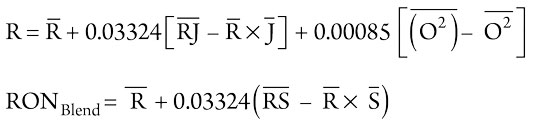(51), (52)

where: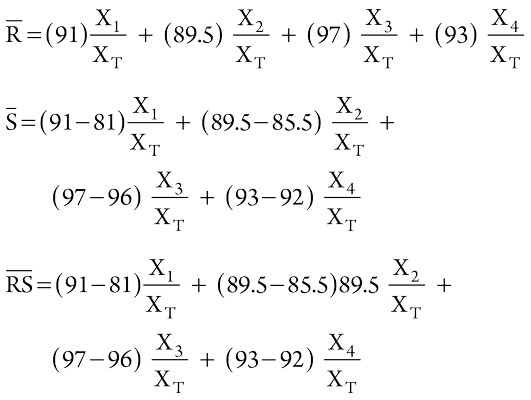(53), (54), (55)

X1 ≥ 0, X2 ≥ 0, X3 ≥ 0 and X4 ≥ 0                                                                                                     (56)

An additional constraint is shown in Eq. 57:

X1 + X2 + X3 + X4 < 15,000 barrels                                                                                                   (57)

The constraint equation for the blend RVP can be obtained by calculating the RVP index from Eq. 58:(58)

where:

BIRVPi is the RVP blending index for component i and RVPi is the RVP of component i in psi. Using the index, the RVP of a blend is (Eqs. 59–61):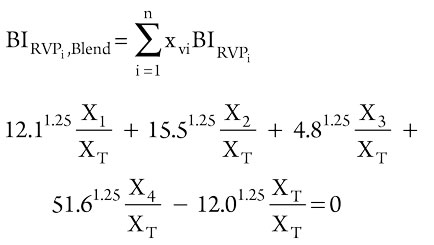(59), (60)

or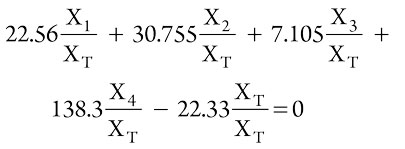(61)

Using the solver in an Excel spreadsheet to maximize RON, the objective function of Eq. 52 with the above constraints (Eqs. 56, 57 and 60), the results are:

RON = 96.54 and X1 = 0, X2 = 0, X3 = 13,259 and
X4 = 1,741 bbl.

If a constraint for FCC gasoline (6,000 barrels) is added, the results for the maximum blend storage would be:

RON= 93.23 and X1 = 0, X2 = 8,855, X3 = 6,000 and
X4 = 145 bbl.

## Case study.9,10

An oil refinery produces a stream of hydrocarbon-based fuel from each of four different processing units. The processing units have capacities of C1, C2, C3 and C4 bpd, respectively. A portion of each base fuel is fed to a central blending station where the base fuels are mixed into three grades of gasoline. The remainder of each base fuel is sold “as is” at the refinery. Storage facilities are available at the blending station for temporary storage of the blended gasolines, if required.

Let N1, N2, N3 and N4 be the octane ratings of the respective base fuels, and let S1, S2, S3 and S4 be the profit derived from their direct sale. Let O1, O2 and O3 be the octane numbers of the three grades of gasoline that must be blended on any given day to satisfy customer demands D1, D2 and D3. Let R1, R2 and R3 be the minimum octane requirements of the three grades of blended gasoline, and let P1, P2 and P3 be the profit per gallon that is realized from the sale of each grade of blended gasoline. Finally, let xij be the quantity of the ith base fuel used to blend the jth gasoline.

The octane number of each gasoline can be expressed as the weighted average of the octane numbers of the constituent base fuels. The weighting factors are the fractions of the base fuels in each gasoline. Therefore (Eqs. 62–64):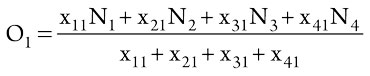(62)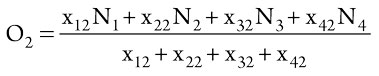(63)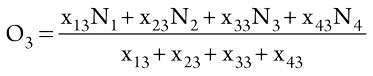(64)

Develop a linear programming model to determine how much of each base fuel should be blended into gasoline and how much should be sold “as is” to maximize profit. Solve the model using numerical data in TABLE 4 and below:

Maximize y = P1 (x11 + x21 + x31 + x41) + P2 (x12 + x22 + x32 + x42)
+ P3 (x13 + x23 + x33 + x43) + (S1/42) (42C1 – x11 – x12 – x13)
+ (S2/42) (42C2 – x21 – x22 – x23) + (S3/42)
(42C3 – x31 – x32 – x33)
+ (S4/42) (42C4 – x41 – x42 – x43)

Subject to ∑ xij ≥ 42Dj

∑ xij Ni x11N1 + x21N2 + x31N3 + x41N4 ≥ R1 (x11 + x21 + x31 + x41)
x12N1 + x22N2 + x32N3 + x42N4 ≥ R2 (x12 + x22 + x32 + x42)
x13N1 + x23N2 + x33N3 + x43N4 ≥ R3 (x13 + x23 + x33 + x43)

where xij = gallons of base fuel i used in gasoline j.

The model assumes full capacity production. The optimal value for the target cell is determined using a what-if analysis tool in the solver, which works by changing the values for parameters that are used to calculate the value of the target cell. The results of the optimization are:

ymax = 111,836,36 at x11 = 436,800, x41= 109,200, x12 = 756,000, x42 = 294,000
x13 = 423,360, x43= 332,640, all other xij = 0.
All xij expressed in terms of gal/d.

The same approach can be used to determine the optimum value of the target parameter when there are changes in other parameters, such as an increase or decrease in selling prices of one and/or all gasoline blends. The same calculation approach can solve for any changes in capacity (C), demand (D) or any other relevant parameter(s).

Alsuhaibani11 employed a linear optimization programming method (LINGO) for the case study. TABLE 5 shows the code and results and are in good agreement with the results obtained from the Excel spreadsheet.12

## Takeaway

Linear programming includes two kinds of programs: a solver program and a mixed inter linear program (MILP). Microsoft Excel incorporates an NLP solver that operates on the values and formulas of a spreadsheet model. The solver uses the spreadsheet interpreter to evaluate the constraint and objective functions, and approximates derivatives using finite differences. The NLP solution engine for the Excel Solver is the generalized reduced gradient (GRG2) algorithm, and this has successfully been implemented in the examples and case study presented in this article and supported by the LINGO program. HP

NOTES

a AspenTech’s Aspen Blend
b AspenTech’s Aspen PIMS-MBO™
c Honeywell’s EBC™
d Honeywell’s OpenBPC™
e Honeywell’s Blend™
f Honeywell’s Profit Controller™
g Invensys’ ROMeo™
h MINOS, developed by Stanford Systems Optimization Laboratory

LITERATURE CITED

1. Maples, R. E., Petroleum refinery process economics, 2nd Ed., PennWell Corp., January 2000.
2. Healy Jr., W. C., C. W. Maasen and R. T. Peterson, “Predicting octane numbers of multi-component blends,” Report number RT-70, Ethyl Corp., Detroit, Michigan, April 1959.
3. Gary, J. H., G. E. Handwerk and M. J. Kaiser, Petroleum refining: Technology and economics, 5th Ed., CRC Press, Taylor & Francis Group, 2007.
4. Jia, Z. and M. Ieraptetritou, “Mixed-integer linear programming model for gasoline blending and distribution scheduling,” Industrial & Engineering Chemistry Research, February 2003.
5. Moro, L., A. Zanin and J. Pinto, “A planning model for refinery diesel production,” Computers & Chemical Engineering, March 1998.
6. Pinto, J. M., M. Joly and L. F. L., “Planning and scheduling models for refinery operations,” Computers & Chemical Engineering, October 2000.
7. Neiro, S. and J. Pinto, “Multiperiod optimization for production planning of petroleum refineries,” Chemical Engineering Communications, Vol. 192, Iss. 1, 2005.
8. Hamisu, A. A., “Petroleum refinery scheduling with consideration for uncertainty,” PhD Thesis, Cranfield University, Cranfield, England, 2015.
9. Aronofsky, J. S., “Linear programming—A problem-solving tool for petroleum industry management,” Journal of Petroleum Technology, July 1962.
10. Gottfried, B. S., Spreadsheet tools for engineers: Excel 5.0 version, McGraw-Hill College, March 1996.
11. Alsuhaibani, A. S., Texas A & M University, private communications.
12. Jiang, S., “Optimization of diesel and gasoline blending operations,” PhD thesis, Centre for Process Integration, School of Chemical Engineering and Analytical Science, The University of Manchester, 2016.

## From the Archive